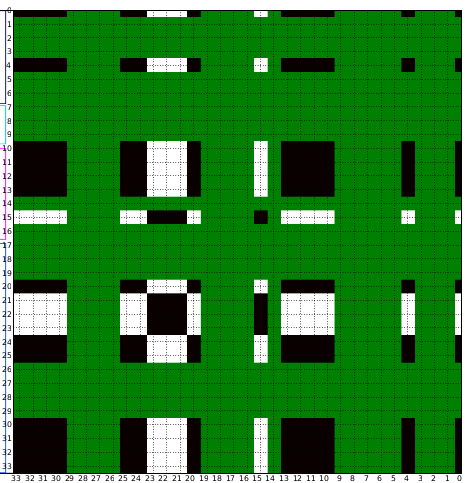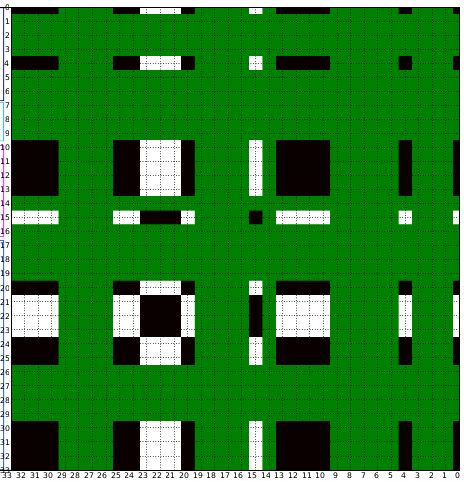# one color per grid: heatmap

Hi,

I am trying to plot a heat map using imshow. My data has 34 rows and 34 columns, so lower limit is 0 and upper is 33, but when I give these limits, it partially removes some of the cells (see 33.png), when I give the limits from 0 to 34, there is an extra white space inserted in the heat map which isn’t part of my data(see 34.png), my next try was to set limits from 0 to 33.5 which sort of solves this problem as the previously concealed colors are now revealed(see 33.5.png), but now I see unqual grid cells on the boundaries of the map (they are almost half the size of the grids cells within the map).

My question is if my data has exactly 34 columns and 34 rows, why do I need an extra 0.5 to do this. Secondly why is that one grid square encompasses 2-3 colors, shouldn’t it just be one color per cell of the grid, which is what I want to see in the map, how can I set the grid lines in such a manner that one color appears per grid cell- is this because I use imshow?

Here is a snippet of my code:

fig = plt.figure(figsize=(25,25))
cmap.set_over(‘green’)
cmap.set_under(‘grey’)
gs=gridspec.GridSpec(1, 2,height_ratios=[1,1,-2,2] ,width_ratios=[1,1,-2,2],hspace=0,wspace=0) phyl_ax=plt.subplot(gs)

ht_ax=plt.subplot(gs)
ht_ax.set_xlim(34,0) ## tried (33.5-0) and (33-0)
ht_ax.set_ylim(34,0) ## tried (33.5-0) and (33-0)
ht_ax.grid(True, which=‘both’)
xticks=range(34)
ht_ax.xaxis.set_ticks(xticks)

ht_ax.yaxis.set_ticks(xticks)
for tick in ht_ax.xaxis.get_major_ticks():
tick.label1.set_horizontalalignment(‘right’)
img = ht_ax.imshow(data, cmap=cmap, interpolation=‘nearest’,vmax=threshold,aspect=‘auto’)

phyl_ax.imshow(img,interpolation=‘bilinear’,aspect=‘auto’)

Note that ht_ax is what I use to draw the heat map

Does anyone see where I am going wrong?

Appreciate any help provided in advance!!

–Asma